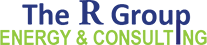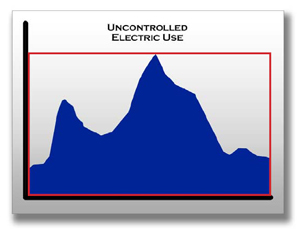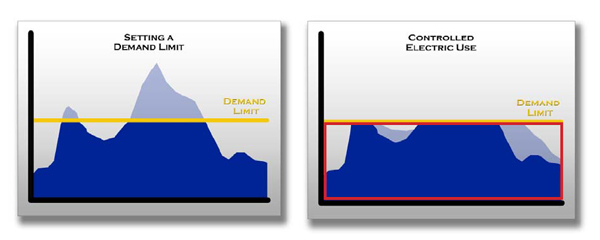## 855.700.7771

Load factor means efficiency. It is the ratio of actual kilowatt-hours used in a given period to the total possible kilowatt -hours that could have been used in the same period, at the peak kW level established by the customer during the billing period.

A high load factor is “a good thing,” and a low load factor is a “bad thing.” A low load factor means that you are using electricity inefficiently relative to what you could be if you were controlling your peak demand.

Load factor is calculated using a few simple numbers from the electric bill. The information required is:

·         Actual kilowatt-hours used during the billing period, in kwh:

·         The Peak kilowatt demand, in kW:

·         The number of days in the billing period:

The ratio that the load factor formula expresses is the comparison between the actual kilowatt-hours used to the total possible kilowatt-hours that could be used at the particular kW level. kWh
——————————— = LF
kW x Days x 24
In the diagram, the red box represents the total possible kilowatt-hours that could be used determined by the peak of electric use. The blue area represents the kilowatt-hours actually used over the month (daily profile shown to simplify drawing). The unshaded area represents the wasted capacity—the area where energy could have been used, but wasn’t. The point is that you paid for the capacity (demand) of the entire box (the demand charge), but didn’t use a large portion of it. Everything in the unshaded area is capacity you paid for but didn’t use.Conceptually, you can think of demand control as making the total size of the box smaller by decreasing the height. In the figures below, the yellow line is the demand limit or set point. By using an Energy Sentry demand controller, the peak demand is reduced by load management, and the original peak of energy use is redistributed below the limit. It’s not about changing how much electricity is used, but when it is used.Using Load Factor to Determine Demand Limit

To determine a kW demand limit for a percentage load factor desired, take the actual kWh’s used by a home in a given month and divide by 720 (total hours in an average 30-day month):

3000 kWh divided by 720 hours = 4.16 (demand limit if at 100% load factor)

If a 60% load factor is desired, take the 4.16 (100% load factor) and divide by .60.

4.16 divided by .60 = ~7kW

If the kW peak is known and the kWh is known, load factor can be found by multiplying the kW by total hours, and dividing the actual kWh’s into that number. For example:

20kW multiplied by 720 hours = 14,400 Total kWh (if at 100% load factor)

3000 kWh divided by 14,400 Total kWh = 21% load factor at 20kW

Recommended Maximum Demand Limits (Typical Residential Application)

 Highest Monthly Usage Hours/Month Load Factor Desired Demand Limit 8000 ÷ by 720 ÷ by 60% = 18.5 7000 ÷ by 720 ÷ by 60% = 16 6000 ÷ by 720 ÷ by 60% = 14 5000 ÷ by 720 ÷ by 60% = 11.5 5000 ÷ by 720 ÷ by 80% = 8.5 4000 ÷ by 720 ÷ by 60% = 9.5 4000 ÷ by 720 ÷ by 80% = 7 3000 ÷ by 720 ÷ by 60% = 7 2000 ÷ by 720 ÷ by 60% = 4.5

Note: The demand limit may be higher or lower due to the individual lifestyle or to extreme variations in the weather.

Calculating Load Factor with Time-Of-Use Rates

If you are working with TOU rates, load factor must be calculated in a different way. TOU rates have separate On-Peak and Off-Peak times which must be calculated separately. The only changes here are:

1. To know how many hours during the billing period were On-Peak and the kilowatt-hours used during this time.
2. The Off-Peak hours during the billing period and the kWh’s used during this time.

If the Peak was different between On and Off-Peak times, use the appropriate Peak kW and use the basic load factor calculation above.

If the TOU rate that you’re dealing with does not bill for demand in the Off-Peak periods, then calculating Off-Peak load factor is not necessary.

Example

Let’s suppose that you are in a situation where:

·         The Time-of-Use Demand rate winter schedule is On-Peak from 7:00 AM to 12:00 PM, a total of 5 hours, Monday through Friday

·         There are 31 days in the month

·         9 weekend (Off -Peak Days), leaving 22 On-Peak days

·         744 hours in the period

·         110 On-Peak hours (15%) and 634 Off-Peak hours (85%)

To calculate the On-Peak load factor, simply take the energy used during the On-Peak times and use the load factor calculation. Let’s say that a total of 4,000 kilowatt-hours were used during the billing period. We’ll assume that 15% or 600 kwh’s were used On-Peak with a demand of 8kW. The load factor is:

600
LF = —————— = 68%
8 x 110

So, roughly two-thirds of the total energy (kwh’s) that could be used during the billing period are in those 22 five hour periods.

Three notes of interest:

1.      Off -Peak load factors will be lower because they have more hours. These are the hours when there’s the lowest average use per hour.

2.      Load factors for On-Peak periods will be higher for shorter on-peak periods. Lower demand limits may be achieved for shorter periods of time. Since the On-Peak Period in the winter is only 5 hours long, a large amount of kilowatt-hour use can be avoided, making it very easy to achieve a lower demand limit.

3.      Holidays that are designated by the electric rate as Off-Peak days in the Off-Peak hours are also included. In the example above, there’s a holiday, bringing the Off-Peak days to 10 and On-Peak days to 21.